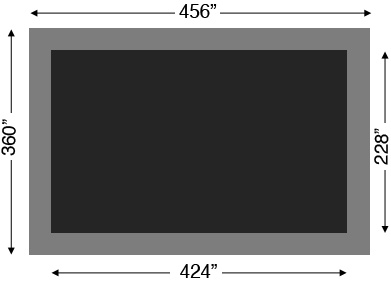SEARCH HOMEMath Central Quandaries & QueriesQuestion from Jeremy, a student: Hello, I'm going to be backfilling a rectangular hole for a shop foundation and need to know how much concrete and gravel I'm going to need to fill it in. The shop foundation is 38 feet wide by 30 feet long and 4 feet deep, there is also a concrete perimeter going inside the foundation that measures 16 inches wide and goes all the way around the inside of the foundationHi Jeremy,

I drew a diagram of the top of the foundation (not to scale) and converted the dimensions to inches.I would first calculate the amount of gravel you need. The gravel fills a space that is 424 inches by 228 inches by 4 feet. You can use our volume calculator to calculate the volume in cubic yards. To find the volume of concrete you need I would use the volume calculator again calculate the volume of the entire region (38 feet by 30 feet by 4 feet) in cubic yards and then subtract the volume of the gravel used.

Write back if you need more assistance,
PennyMath Central is supported by the University of Regina and the Imperial Oil Foundation.